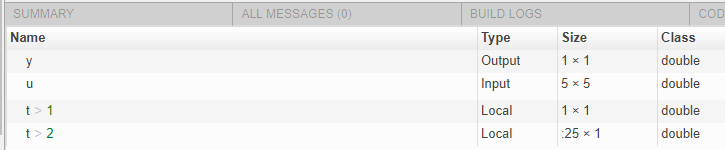## Reuse the Same Variable with Different Properties

For C/C++ code generation, there are certain variables that you can reassign after the initial assignment with a value of different class, size, or complexity. A variable can hold values that have the same class and complexity but different sizes. If the size of the initial assignment is not constant, the variable is dynamically sized in generated code. For more information, see Variable-Size Data.

You can reassign the type (class, size, and complexity) of a variable after the initial assignment if each occurrence of the variable can have only one type. In this case, the variable is renamed in the generated code to create multiple independent variables.

### When You Can Reuse the Same Variable with Different Properties

You can reuse (reassign) an input, output, or local variable with different class, size, or complexity if the code generator can unambiguously determine the properties of each occurrence of this variable during C/C++ code generation. If so, MATLAB® creates separate uniquely named local variables in the generated code. You can view these renamed variables in the code generation report.

A common example of variable reuse is in `if-elseif-else` or `switch-case` statements. For example, the following function `example1` first uses the variable t in an `if` statement, where it holds a scalar double, then reuses t outside the `if` statement to hold a vector of doubles.

```function y = example1(u) %#codegen if all(all(u>0)) % First, t is used to hold a scalar double value t = mean(mean(u)) / numel(u); u = u - t; end % t is reused to hold a vector of doubles t = find(u > 0); y = sum(u(t(2:end-1)));```

### When You Cannot Reuse Variables

You cannot reuse (reassign) variables if it is not possible to determine the class, size, and complexity of an occurrence of a variable unambiguously during code generation. In this case, variables cannot be renamed and a compilation error occurs.

For example, the following `example2` function assigns a fixed-point value to x in the `if` statement and reuses x to store a matrix of doubles in the `else` clause. It then uses x after the `if-else` statement. This function generates a compilation error because after the `if-else` statement, variable x can have different properties depending on which `if-else` clause executes.

```function y = example2(use_fixpoint, data) %#codegen if use_fixpoint % x is fixed-point x = fi(data, 1, 12, 3); else % x is a matrix of doubles x = data; end % When x is reused here, it is not possible to determine its % class, size, and complexity t = sum(sum(x)); y = t > 0; end ```

Example 3. Variable Reuse in an if Statement

To see how MATLAB renames a reused variable `t`:

1. Create a MATLAB file `example1.m` containing the following code.

```function y = example1(u) %#codegen if all(all(u>0)) % First, t is used to hold a scalar double value t = mean(mean(u)) / numel(u); u = u - t; end % t is reused to hold a vector of doubles t = find(u > 0); y = sum(u(t(2:end-1))); end```

2. Generate a MEX function for `example1` and produce a code generation report.

`codegen -o example1x -report example1.m -args {ones(5,5)}`

3. Open the code generation report.

On the Variables tab, you see two uniquely named local variables `t>1` and `t>2`.4. In the list of variables, click `t>1`. The report highlights the instances of the variable `t` that are inside of the `if` statement. These instances of `t` are scalar double.

5. Click `t>2`. The code generation report highlights the instances of `t` that are outside of the `if` statement. These instances of `t` are variable-size column vectors with an upper bound of `25`.

### Limitations of Variable Reuse

The following variables cannot be renamed in generated code:

• Persistent variables.

• Global variables.

• Variables passed to C code using `coder.ref`, `coder.rref`, `coder.wref`.

• Variables whose size is set using `coder.varsize`.

• Variables whose names are controlled using `coder.cstructname`, when generating code by using MATLAB Coder™.

• The index variable of a `for`-loop when it is used inside the loop body.

• The block outputs of a MATLAB Function block in a Simulink® model.

• Chart-owned variables of a MATLAB function in a Stateflow® chart.